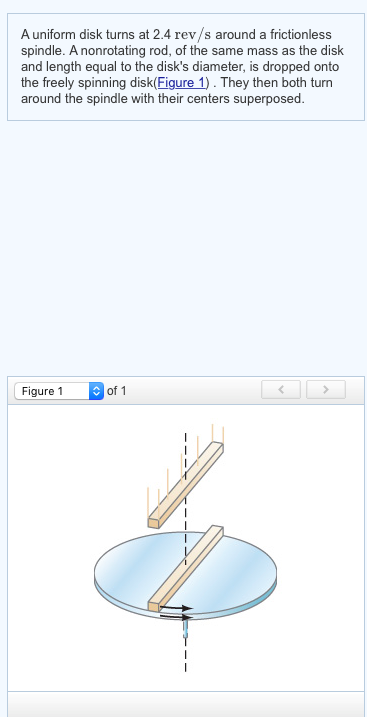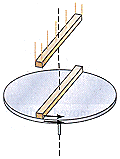# A uniform disk turns at 2.7 rev/s around a frictionless central axis. A nonrotating rod, of the same mass as the disk a...

A uniform disk turns at 2.7 rev/s around a frictionless central axis. A nonrotating rod, of the same mass as the disk and length equal to the disk's diameter, is dropped onto the freely spinning disk(Figure 1) . They then turn around the spindle with their centers superposed.
What is the angular frequency in rev/s of the combination?

using law of conservation of angular momentum

Idisk * wdisk = (Idisk + Irod) * wf

wf = Idisk * wdisk / (Idisk + Irod)

we know that

Idisk = m*r^2 / 2

Irod = m*L^2 / 12 = m*4r^2 / 12

wf = (wdisk * m*r^2/2) / m*r^2/2 + m*4r^2/12

wf = (wdisk / 2) / (1/2 + 1/3)

wf = o.60 * wdisk

wf = 0.60 * 2.7

wf = 1.62 rev/s

##### Add Answer of: A uniform disk turns at 2.7 rev/s around a frictionless central axis. A nonrotating rod, of the same mass as the disk a...
Similar Homework Help Questions
• ### A uniform disk turns at 3.6 rev/s around a frictionless central axis. A nonrotating rod, of the same mass as the dis...

A uniform disk turns at 3.6 rev/s around a frictionless central axis. A nonrotating rod, of the same mass as the disk and length equal to the disk's diameter, is dropped onto the freely spinning disk(Figure 1). They then turn around the spindle with their centers superposed. Figure 1 of 1 Part A What is the angular frequency in rev/s of the combination? # - - - -

• ### A uniform disk turns at 3.7 rev/s around a frictionless spindle. A nonrotating rod, of the same mass as the disk and len...

A uniform disk turns at 3.7 rev/s around a frictionless spindle. A nonrotating rod, of the same mass as the disk and length equal to the disk's diameter, is dropped onto the freely spinning disk. They then turn together around the spindle with their centers superposed. What is the angular frequency in rev/s of the combination?

• ### What is the angular frequency in rev/s of the combination? A uniform disk turns at 2.4 rev/s around a frictionless sp...What is the angular frequency in rev/s of the combination? A uniform disk turns at 2.4 rev/s around a frictionless spindle. A nonrotating rod, of the same mass as the disk and length equal to the disk's diameter, is dropped onto the freely spinning disk(Figure 1). They then both turn around the spindle with their centers superposed. Figure 1 of 1

• ### A uniform disk turns at 3.6 rev/s around a frictionless spindle. A non rotating rod, of the same mass as the disk and l...

A uniform disk turns at 3.6 rev/s around a frictionless spindle. A non rotating rod, of the same mass as the disk and length equal to the disk's diameter, is dropped onto the freely spinning disk . They then both turn around the spindle with their centers superposed. What is the angular frequency in rev/s of the combination? please express answer in proper significant figures and rounding.

• ### Angular Frequency problem

A uniform disk turns at 3.7 rev/s around a frictionless spindle. A nonrotating rod, of the same mass as the disk and length equal to the disk's diameter, is droppedonto the freely spinning disk. They then turn together around the spindle with their centers superposed. What is the angular frequency in rev/s of the combination?

• ### angular frequencyA uniform disk turnsat 3.6 around a frictionless spindle. A nonrotating rod, of thesame mass as the disk and length equal to the disk's diameter, isdropped onto the freely spinning disk.They then turn togetheraround the spindle with their centers superposed.(Intro 1 figure)What is the angular frequency in of the combination?(Please explain well, thanks)

• ### physics

A uniform disk turns at 1.8 around a frictionless spindle. A nonrotating rod, of the same mass as the disk and length equal to the disk's diameter, is dropped onto thefreely spinning disk . They then both turn around the spindle with their centers superposed

• ### Rotational MotionA uniform disk turns at 9.5 rev/saround a frictionless spindle. A nonrotating rod, of the same massas the disk and length equal to the disk's diameter, is droppedontothe freely spinning disk, see the figure. They then turntogether around the spindle with their centers superposed. What isthe angular velocity of thecombination? rev/sHelp!

• ### Rotational Motion

A uniform disk turns at 5.0 rev/s around a frictionlessspindle. A nonrotating rod, of the same mass as the disk and lengthequal to the disk's diameter, is droppedonto the freely spinningdisk. They then both turn around the spindle with their centerssuperposed. What is the angular frequency in rev/s of thecombination?

• ### Vectors/cross product/angular frequency / conservation of angular momentum.

i)The vector cross product of two vectors A and B is zero. What is the angle between these vectors?Show work.ii)A uniform disk turns at 2.4 rev/sec around a frictionless spindle. A nonrotating rod,of the same mass as thedisk and length equal to the disk diameter is dropped onto the freely spinning disk as shown in the figure.They then both turn around the spindle with theircenters superposed. What is the angular frequency in rev/sec of the combination? {Disk= I=(1/2)MR^2} and {Rod=I=(1/12)ML^2....

Need Online Homework Help?